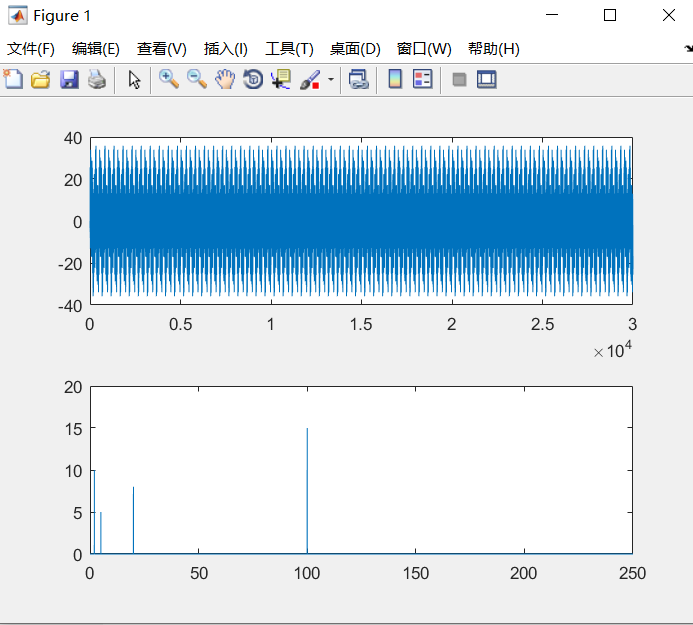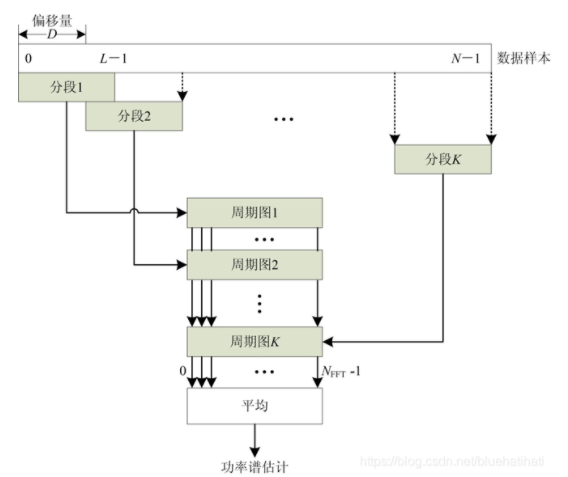﻿ 转载：功率谱学习及matlab代码_qinghuanduji的博客-程序员宝宝_功率谱matlab - 欧菲博客

# 转载：功率谱学习及matlab代码_qinghuanduji的博客-程序员宝宝_功率谱matlab

## 前记

• 只接触很少信号处理的问题，该篇是查阅资料总结的，先对概念等内容进行介绍，最后附matlab的功率谱代码。
• 看了很多资料，没有说明白为啥可以有这么多种方法计算，也不清楚具体这种方法计算出来的是否正确，就写了一篇总结篇总结一下。

## 功率谱与频谱在计算上的区别

• 功率谱：信号自相关后FFT
• 频谱：信号直接FFT

## 能量信号和功率信号的介绍

• 二者的定义
• 能量信号：又称能量有限信号，是指在所有时间上总能量不为零且有限的信号。
• 功率信号：它的能量为无限大，它对通信系统的性能有很大影响，决定了无线系统中发射机的电压和电磁场强度。
• 二者的收敛性
• 能量信号其傅氏变换收敛（即存在），而功率信号傅氏变换通常不收敛。（当然，若信号存在周期性，可引入特殊数学函数（Delta）表征傅氏变换的这种非收敛性）
• 实际信号的介绍
• 实际信号多为随机信号，这类信号的特点是状态随机性随时间无限延伸，其样本能量无限。换句话说，随机信号（样本）大多属于功率信号而非能量信号，它并不存在傅氏变换，亦即不存在频谱
• 实际中我们获得的往往仅仅是信号的一段，此时即使信号为功率信号，截断之后其傅氏变换收敛，但此变换结果严格来讲不属于任何“谱”（进一步分析可知它是样本真实频谱的平滑：卷积谱）；对上述变换若取其幅度平方，可作为平稳信号功率谱（密度）的近似，是为经典的“周期图法
• 随机信号的介绍
• 随机信号又称随机过程，很多噪声属于特殊的随机过程，它们的某些统计特性具有平稳性，其均值和自相关函数具有平稳性。对于这样的随机过程，自相关函数蜕化为一维确定函数，前人证明该确定相关函数存在傅氏变换

## 概念解释

• 功率谱定义及计算
• 一个是自相关函数傅立叶变换；（维纳辛钦定理）
• 另一个是时域信号傅氏变换模平方然后除以时间长度。（来自能量谱密度）
• 信号的传播都是看不见的，但是它以波的形式存在着，这类信号会产生功率，单位频带的信号功率就被称之为功率谱。它可以显示一定区域信号功率随着频率变化的分布情况
• 注：根据parseval定理，信号傅氏变换模平方被定义为能量谱，能量谱密度在时间上平均就得到了功率谱。
• 频谱的定义及计算
• 频谱是频率谱密度的简称，是频率的分布曲线。常常指信号的Fourier变换。

## 两者的联系与区别

• 能量信号频谱通常既含有幅度也含有相位信息
• 幅度谱的平方（二次量纲）又叫能量谱（密度），它描述了信号能量频域分布
• 功率信号的功率谱（密度）描述了信号功率频率的分布特点（密度：单位频率上的功率）。
• 平稳信号功率谱密度恰好是其自相关函数的傅氏变换
• 对于非平稳信号，其自相关函数的时间平均（对时间积分，随时变性消失而再次退变成一维函数）与功率谱密度仍是傅氏变换对
• 一个信号的频谱,只是这个信号从时域表示转变为频域表示,频谱只是同一种信号的不同的表示方式而已功率谱是从能量的观点对信号进行的研究,其实频谱和功率谱的关系归根结底还是信号和功率,能量等之间的关系。

## 谱估计

• 功率谱估计一般分成两大类：
• 经典谱估计，也称为非参数谱估计
• 现代谱估计，也称为参数谱估计

## matlab功率谱估计

• PART 1: 产生原始信号及显示
``````clear;clc;close all;

%% 产生原始信号
f1=5;f2=2;f3=20; f4=100;
fs=500;                  			       % 采样率
N=fs*60;                    			   % 采样点数
t = 0 : 1/fs : (N-1)/fs;       			   % 横坐标t坐标精度
% noise_create= randn(1,length(t));        % 产生含有噪声的序列
y1=5*sin(2*pi*f1*t)+10*sin(2*pi*f2*t)+8*sin(2*pi*f3*t)+15*sin(2*pi*f4*t);
figure(1);subplot(2,1,1);plot(y1,'r');     % 画出原信号的时频图

%% 画出原信号的频率谱
n=0:N-1;
f=n*fs/N;                      			   % 频谱图的频率序列
y=fft(y1,N);                  			   % 对信号进行快速傅里叶变换
mag=abs(y)*2/N;                			   % 求得傅里叶变换后的振幅，与真实幅值相比需要做abs(y)*2/N的运算
subplot(2,1,2); plot(f(1:N/2),mag(1:N/2)); % 绘出Nyquist频率之前随频率变化的振幅
``````• PART 2: 直接法(周期图法)求功率谱
``````%%  直接法(周期图法)求功率谱
% 直接将信号的采样数据x(n)进行Fourier变换求取功率谱密度估计的方法
nfft=length(y1);                           % 采样的点数
window=boxcar(length(y1));                 % 使用矩形窗(默认为矩形窗，窗的大小与信号长度一样)
[Pxx,f]=periodogram(y1,window,nfft,fs);    % 直接法
figure(2);subplot(2,2,1);plot(y1);title('信号波形');
subplot(2,2,2);plot(f,10*log10(Pxx));title('周期图法原始信号的功率谱');  %绘制分贝形式的功率谱
% pxx和fft后的平方是一个量纲(w/hz)，10*log10就是用db做单位。

``````
• PART 3: 间接法求功率谱(先估计出自相关函数，再傅里叶变化求功率谱)
``````%%  间接法求功率谱(先估计出自相关函数，再傅里叶变化求功率谱)
nfft=length(y1);
cxn=xcorr(y1,'unbiased');                  % 计算序列的自相关函数
CXk=fft(cxn,nfft);
Pxx=abs(CXk);
index=0:round(nfft/2-1);
k=index*fs/nfft;
plot_Pxx=10*log10(Pxx(index+1));
subplot(2,2,3);plot(y1);title('信号波形');
subplot(2,2,4);plot(k,plot_Pxx);title('间接法原始信号的功率谱');     %绘制分贝形式的功率谱
``````
• PART 4: 改进直接法：Bartlett法
``````%% 改进直接法：Bartlett法（将N点的有限长序列x(n)分段求周期图再平均。）
% 对于直接法的功率谱估计，当数据长度N太大时，谱曲线起伏加剧，若N太小，谱的分辨率又不好，因此需要改进。
nfft=length(y1);
window=boxcar(length(y1));                              %矩形窗
noverlap=0;                                            %数据无重叠
[Pxx,Pxxc]=spectrogram(y1,window,noverlap,nfft,fs);
index=0:round(nfft/2-1);
k=index*fs/nfft;
plot_Pxx=10*log10(Pxx(index+1));
plot_Pxxc=10*log10(Pxxc(index+1));
figure(4);
subplot(1,2,1);
plot(k,plot_Pxx);title('直接法信号');
subplot(1,2,2);
plot(k,[plot_Pxx plot_Pxx-plot_Pxxc plot_Pxx+plot_Pxxc]);title('Bartlett信号');
``````
• PART 5: 加窗平均周期图法 Welch法
``````%% 加窗平均周期图法 Welch法（选择适当的窗函数，并在周期图计算前直接加进去）
% % Welch法对Bartlett法进行了两方面的修正，一是选择适当的窗函数w(n)w(n)w(n)，并再周期图计算前直接加进去，
% % 加窗的优点是无论什么样的窗函数均可使谱估计非负。二是在分段时，可使各段之间有重叠，这样会使方差减小。
Fs=500;
n=0:1/Fs:1;
nfft=length(y1);
window=boxcar(N);                                         % 矩形窗（时间变量的零次幂窗）
window1=hamming(N);                                       % 海明窗（改进的升余弦窗）
window2=blackman(N);                                      % blackman窗
noverlap=0;                                               % 数据无重叠
range='onesided';                                         % 频率间隔为[0 Fs/2]，只计算一半的频率
[Pxx,f]=pwelch(y1,window,noverlap,nfft,Fs,range);
[Pxx1,f1]=pwelch(y1,window1,noverlap,nfft,Fs,range);
[Pxx2,f2]=pwelch(y1,window2,noverlap,nfft,Fs,range);
plot_Pxx=10*log10(Pxx);
plot_Pxx1=10*log10(Pxx1);
plot_Pxx2=10*log10(Pxx2);
figure(5);
subplot(1,3,1);
plot(f,plot_Pxx);title('矩形窗');                         % 主瓣比较集中，缺点是旁瓣较高，并有负旁瓣，导致变换中带进了高频干扰和泄漏，甚至出现负谱现象。

subplot(1,3,2);                                           % 海明窗的频谱也是由3个矩形时窗的频谱合成，但其旁瓣衰减速度为20dB/（10oct）
plot(f1,plot_Pxx1);title('汉明窗');                       % (改善频率泄露)加上汉明窗，只有中间的数据体现出来了，两边的数据信息丢失了，所以等会移窗的时候，只会移1/3或1/2窗，这样被前一帧或二帧丢失的数据又重新得到了体现。

subplot(1,3,3);
plot(f2,plot_Pxx2);title('blackman窗');
``````

## 注释

• [Pxx,f] = periodogram(x,window,nfft,fs)的解释：
• window：所使用的窗口，默认是boxcar（矩形窗），其长度必须与x的长度一致；
• nfft(傅里叶变换长度)：采样点数。
• fs：采样频率。
• [S,F,T,P]=spectrogram(x,window,noverlap,nfft,fs)
[S,F,T,P]=spectrogram(x,window,noverlap,F,fs)的解释：
• x—输入信号的向量。默认情况下，即没有后续输入参数，x将被分成8段分别做变换处理，如果x不能被平分成8段，则会做截断处理。
• window—窗函数，默认为nfft长度的海明窗Hamming
• noverlap—每一段的重叠样本数，默认值是在各段之间产生50%的重叠。
• nfft—做FFT变换的长度，默认为256和大于每段长度的最小2次幂之间的最大值。另外，此参数除了使用一个常量外，还可以指定一个频率向量F
• fs—采样频率，默认值归一化频率。
• 周期图法改进——Bartlett方法和Welch方法
• Bartlett方法：D=L； Welch方法： D=L/2
• Bartlett方法就是把数据分D段，每段fft模平方除以每段长度，再把D段的s相加再平均。
• Welch方法就是有重复的分段，具体如下图：## 能量谱的介绍与代码

• 能量谱也叫能量谱密度，能量谱密度描述了信号或时间序列的能量如何随频率分布。能量谱是原信号傅立叶变换的平方
• 下面是之前的一个代码（不确定是否正确）
``````y=fft(y1,N);
A1=abs(y1);
A2=A1.^2;
``````

## 补充：

1. 功率谱密度的单位是什么，dB与W/Hz的介绍
• 功率谱的单位是W/Hz，单位是dB时是做了对数处理（10logX）。
• 取对数的目的是使那些振幅较低的成分相对高振幅成分得以拉高，以便观察掩盖在低幅噪声中的周期信号
2. 求功率谱的两种方法哪个好？
• 从原理上讲似乎没什么区别，从MATLAB的结果上来看，相关函数法对噪声的抑制效果更好，图线更平滑。
3. FFT和PSD都是表示的频谱特性，帮助我们找出峰值的位置，那么有了FFT为什么还要提出PSD
• 信号分为确定信号随机信号，而确定信号又分为能量信号功率信号随机信号一定是功率信号
• 根据狄里赫利条件，能量信号可以直接进行傅里叶变换，而功率信号不行
• 对于无法做傅里叶变换的信号，只能走一步弯路，先求自相关，再做傅里叶。但是物理意义上就是功率谱了。不过总之得到了信号的频率特性。
4. 既然为什么随机信号的一次FFT没有意义却还能(傅立叶变换的平方)/(区间长度)得到功率谱？
• 对随机信号直接做FFT的做法其实就是截断成能量信号进行处理，这种处理不符合随机信号定义，但之所以这样做，是做短时频域分析下作的近似处理。
5. 频谱和能量谱（也叫能量谱密度）是傅里叶变换得到的复数结果和模平方的关系； 而功率谱（也就是功率谱密度）是针对随机信号分析提出的概念。